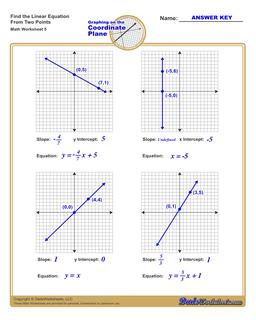PLEASE GO BACK AND USE THE BIG BLUE 'PRINT' BUTTON ON THE PAGE TO PRINT THE WORKSHEET CORRECTLY!Sorry for the trouble! The browser won't print the embedded worksheet PDF directly using the normal 'Print' command in the file menu, so you need to click the big 'Print' button to send just the worksheet and not the surrounding page to the printer.

# Math Worksheets: Linear Equations: Linear Equations: Find the Equation of a Line## Find the Equation of a Line

Property Value
DescriptionFind the Equation of a Line: Each linear equations worksheet on this page shows four graphs on a coordinate plane, each with two points labeled, and students find the equation in slope-intercept form by calculating both the slope and y-intercept.
Resource TypeWorksheet